Courses

# Factors that Affect Solubility Class 12 Notes | EduRev

## Chemistry Class 12

Created by: Mohit Rajpoot

## JEE : Factors that Affect Solubility Class 12 Notes | EduRev

The document Factors that Affect Solubility Class 12 Notes | EduRev is a part of the JEE Course Chemistry Class 12.
All you need of JEE at this link: JEE

EFFECT OF PRESSURE ON SOLUBILITY OF GASES IN LIQUIDS: HENRY’S LAW
Henry’s Law gives a quantitative relation between pressure and gas solubility in a liquid.
It states that:
“The solubility of a gas in a liquid is directly proportional to the partial pressure of the gas present above the surface of liquid or solution”.
The solubility is taken as the mass of the gas dissolved per unit volume of the liquid. Thus, if m is the mass of the gas dissolved per unit volume of the solvent and P is the pressure of the gas in equilibrium with the solution, then

m ∝ p
m = Kp

where, K is the proportionality constant.
When P = 1, m = K, i. e., the solubility of the gas at unit pressure is equal to constant K.

The magnitude of K depends upon

• The nature of the gas,
• Nature of solvent,
• Temperature and
• The units of pressure.

Above Equation represents equation of straight line (y = mx + c) passing through origin (c = 0)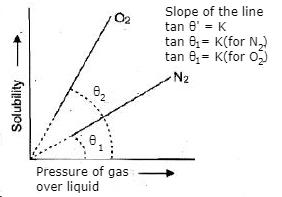This plot shows the variation of solubility of some gases against equilibrium pressure. The straight line graphs show the validity of Henry's law.

Dalton, a contemporary of Henry, also concluded independently that the solubility of a gas in a liquid solution is a function of the partial pressure of the gas. If we use the mole fraction of the gas in the solution as a measure of its solubility, then:

Mole fraction of the gas in a solution is proportional to the partial pressure of the gas.
i.e,
partial pressure of the gas in solution = KH × mole fraction of the gas in solution
P = KH × xgas

Here KH is Henry’s law constant
or,
If we draw a graph between partial pressure of the gas versus mole fraction of the gas in solution, then we should get a plot of the type as shown in figure.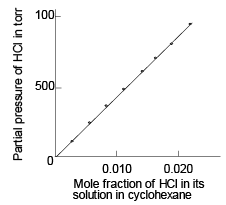Experimental results for the solubility of HCl gas in cyclohexane at 93 K. The slope of the line is the Henry's law constant KH.

• Different gases have different KH values at the same temperature. This suggests that KH is a function of the nature of the gas. Table gives KH values of some common gases at specified temperature. Values of Henry’s law constant (KH) for some selected gases in water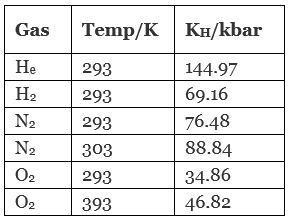• It is obvious from figure that the higher the value of KH at a given pressure, the lower is the solubility of the gas in the liquid.
• It can be seen from table that KH value for both N2 and O2 increases with increase in temperature indicating that solubility of gases decreases with increase of temperature.
• It is due to this reason that aquatic species are more comfortable in cold waters rather than warm waters.

(i) Solubility of a gas in liquid increases with increase in pressure and vice verse. That is solubility of gas in liquid decreases with decrease in pressure.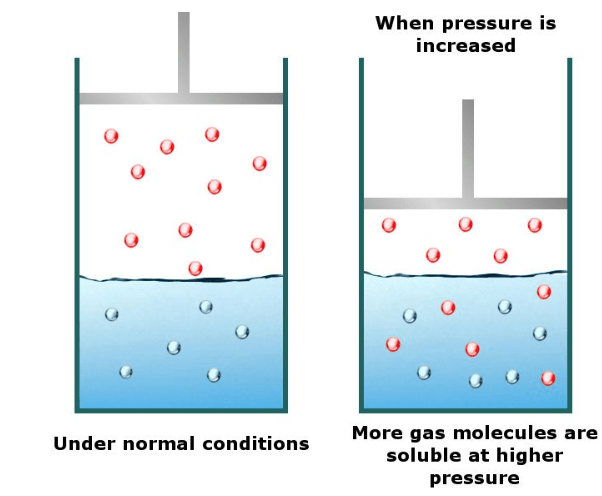Fig: Henry's law

(ii) The solubility of a gas in liquid decreases with increase in Henry’s Law constant (KH), at a given pressure.

This means at a given pressure higher the value of KH (Henry’s Law constant), the lower is the solubility of the gas in a liquid and vice versa.

Example.1. If N2 gas is bubbled through water at 293 K, how many millimoles of N2 gas would dissolve in 1 litre of water? Assume that N2 exerts a partial pressure of 0.987 bar. Given that Henry’s law constant for N2 at 293 K is 76.48 kbar.
Solution.
The solubility of gas is related to the mole fraction in aqueous solution.
The mole fraction of the gas in the solution is calculated by applying Henry’s law. Thus:
x (Nitrogen) = p(nitrogen)/KH = 0.987 bar/76.480 = 1.29 × 10-5
As 1 litre of water contains 55.5 mol of it, therefore if n represents number of moles of N2 in solution,
x (Nitrogen) = n mol/n mol + 55.5 mol = n/55.5 = 1.29 × 10-5
(n in denominator is neglected as it is < < 55.5)
Thus n = 1.29 × 10–5 × 55.5 mol = 7.16 × 10–4 mol

APPLICATION OF HENRY’S LAW
(i) Since solubility of gas in liquid increases with increase in pressure, hence to increase the solubility of CO2 in soft drinks and soda water, the bottle is sealed under high pressure.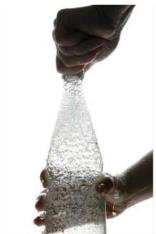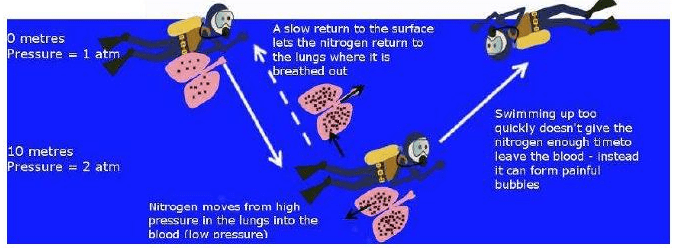Fig: Bends: Medical condition.

(ii) Scuba divers have to face the problems of bends. When they dive and go deeper in water, pressure gradually increases resulting in increase in the solubility of atmospheric gases in blood. Bends is a medical condition which blocks the blood capillaries due to formation of bubbles of nitrogen in blood. Bends is very painful and dangerous to life.
When scuba divers come towards the surface of water they have to face bends. Hence scuba diver use a tank filled with air diluted with helium (11.7% helium, 56.2% nitrogen and 32.1% oxygen) to avoid bends as well as toxic effects of high concentration of nitrogen in the blood because of increase in pressure underwater and decreasing pressure towards the water surface.

(iii) People, who live at high altitudes and climbers (mountaineers) have to face a problem called anoxia. Anoxia is also a medical condition.Fig: Mountaineers using oxygen cylinders.

The partial pressure of oxygen in atmosphere decreases with high altitude. Decrease in partial pressure of oxygen leads to low concentration of oxygen in blood and tissues. Because of low concentration of oxygen people feel short of breath and become weak and unable to think clearly.
To avoid the condition of anoxia, mountaineers use oxygen cylinder while climbing to high altitude.

(iv) In lungs, where oxygen is present in air with high partial pressure, haemoglobin combines with oxygen to form oxyhaemoglobin. In tissues where partial pressure of oxygen is low, oxyhaemoglobin releases oxygen for utilization in cellular activities.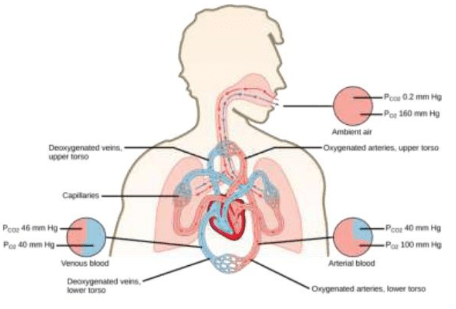Limitations of Henry's Law
Henry's law holds good if the following conditions are fulfilled:

• The pressure is not too high,
• The temperature is not very low,
• The gas does not chemically combine with the solvent.

Example.2. Calculate the concentration of CO2 in a soft drink that is bottled at partial pressure of CO2 of 4 atm' over the liquid at 25° C. The Henry’ Law constant for CO2 in water at 25 ˚C is 3.1 × 10-2 mol/litre-atm.
Solution.
According to Henry's Law:
S = KP
3.1 × 10-2 × 4 = 0.12 mol litre-1

Try Yourself!
Q.1. At 20° C the solubility of nitrogen gas in water is 0.0150 g/litre when the partial pressure N2 is 580 torr. Find the solubility N2 in H2O at 20°C when its partial pressure is 800 torr.
Ans. 0.0207 g/litre.

Offer running on EduRev: Apply code STAYHOME200 to get INR 200 off on our premium plan EduRev Infinity!

,

,

,

,

,

,

,

,

,

,

,

,

,

,

,

,

,

,

,

,

,

;